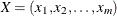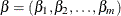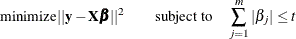# Shared Statistical Concepts

#### Lasso Selection

Method=LASSO specifies the least absolute shrinkage and selection operator (LASSO) method, which is supported in the HPREG procedure. LASSO arises from a constrained form of ordinary least squares regression where the sum of the absolute values of the regression coefficients is constrained to be smaller than a specified parameter. More precisely letdenote the matrix of covariates and let y denote the response, where thes have been centered and scaled to have unit standard deviation and mean zero and y has mean zero. Then for a given parameter t, the LASSO regression coefficientsare the solution to the following constrained optimization problem:Provided that the LASSO parameter t is small enough, some of the regression coefficients are exactly 0. Hence, you can view the LASSO as selecting a subset of the regression coefficients for each LASSO parameter. By increasing the LASSO parameter in discrete steps, you obtain a sequence of regression coefficients in which the nonzero coefficients at each step correspond to selected parameters.

Early implementations (Tibshirani 1996) of LASSO selection used quadratic programming techniques to solve the constrained least squares problem for each LASSO parameter of interest. Later Osborne, Presnell, and Turlach (2000) developed a "homotopy method" that generates the LASSO solutions for all values of t. Efron et al. (2004) derived a variant of their algorithm for least angle regression that can be used to obtain a sequence of LASSO solutions from which all other LASSO solutions can be obtained by linear interpolation. This algorithm for METHOD= LASSO is used in PROC HPREG. It can be viewed as a stepwise procedure with a single addition to or deletion from the set of nonzero regression coefficients at any step.

As in the other selection methods that are supported by high-performance statistical procedures, you can use the CHOOSE= option to specify a criterion to choose among the models at each step of the LASSO algorithm. You can also use the STOP= option to specify a stopping criterion. For more information, see the discussion in the section Forward Selection. The model degrees of freedom that PROC GLMSELECT uses at any step of the LASSO are simply the number of nonzero regression coefficients in the model at that step. Efron et al. (2004) cite empirical evidence for doing this but do not give any mathematical justification for this choice.

A modification of LASSO selection suggested in Efron et al. (2004) uses the LASSO algorithm to select the set of covariates in the model at any step, but it uses ordinary least squares regression and just these covariates to obtain the regression coefficients. You can request this hybrid method by specifying the LSCOEFFS suboption of SELECTION= LASSO.